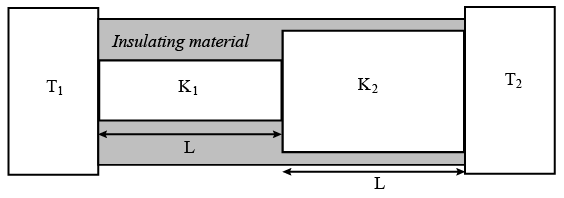Instructions

For each question, enter the correct numerical value (in decimal notation, truncated/rounded-off to the second decimal place; e.g. 6.25, 7.00, -0.33, -.30, 30.27, -127.30) using the mouse and the on-screen virtual numeric keypad in the place designated to enter the answer.

Question 11

Backspace
789
456
123
0.-
Clear All
Question 12

Backspace
789
456
123
0.-
Clear All
Question 13

Backspace
789
456
123
0.-
Clear All
Question 14

# Two conducting cylinders of equal length but different radii are connected in series between two heat baths kept at temperatures $$𝑇_{1} = 300 𝐾 and 𝑇_{2}$$ = 100 𝐾, as shown in the figure. The radius of the bigger cylinder is twice that of the smaller one and the thermal conductivities of the materials of the smaller and the larger cylinders are $$𝐾_{1} and 𝐾_{2}$$ respectively. If the temperature at the junction of the two cylinders in the steady state is 200 𝐾, then $$\frac{𝐾_{1}}{𝐾_{2}}$$ =__________.Backspace
789
456
123
0.-
Clear All
Instructions

In electromagnetic theory, the electric and magnetic phenomena are related to each other. Therefore, the dimensions of electric and magnetic quantities must also be related to each other. In the questions below, [E] and [B] stand for dimensions of electric and magnetic fields respectively, while $$[\epsilon_{0}]$$ and $$[\mu_{0}]$$ stand for dimensions of the permittivity and permeability of free space respectively. [L] and [T] are dimensions of length and time respectively. All the quantities are given in SI units.

Question 15

Question 16

# The relation between $$[\epsilon_{0}]$$ and $$[\mu_{0}]$$ is

Instructions

If the measurement errors in all the independent quantities are known, then it is possible to determine the error in any dependent quantity. This is done by the use of series expansion and truncating the expansion at the first power of the error. For example, consider the relation $$Z = \frac{x}{y}$$. If the errors in x, y and z are $$\triangle x, \triangle y$$ and $$\triangle z$$ respectively, then $$Z \pm \triangle Z = \frac{x \pm \triangle x}{y \pm \triangle y}=\frac{x}{y} \left(1 \pm \frac{\triangle x}{x} \right) \left(1 \pm \frac{\triangle y}{y} \right)^{-1}$$. The series expansion for $$\left(1 \pm \frac{\triangle y}{y}\right)^{-1}$$, to to first power in $$\frac{\triangle y}{y}$$, is $$1 \mp \left(\frac{\triangle y}{y}\right)$$ The relative errors in independent variables are always added. So the error in z will be $$\triangle z = z(\frac{\triangle x}{x} + \frac{\triangle y}{y})$$. The above derivation makes the assumption that $$\frac{\triangle x}{x} \ll 1, \frac{\triangle y}{y} \ll 1$$.Therefore, the higher powers of these quantities are neglected.

Question 17

Question 18

# In an experiment the initial number of radioactive nuclei is 3000. It is found that $$1000 \pm 40$$ nuclei decayed in the first 1.0 s. For $$\mid x \mid \ll 1$$, $$\ln(1 + x)$$ = x up to first power in x. The error $$\triangle \lambda$$, in the determination of the decay constant $$\lambda$$, in $$s^{-1}$$, is , is

Instructions

For the following questions answer them individually

Question 19

Question 20

OR